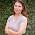### Probablity of NYTBS

I was thinking of a the probability of being a New York Times Best Selling author. I think it's some random equation. Here are the variables:

O=originality
w=weird factor
t=timing
c=connection
l=talent

NYTBS=O*w*1/4t8C/l

Er something

1.So... if I'm looking at that correctly, that can be simplified to:

O*w*t*c*2/l

So... the more talented the writer, the less likely to be a NYTBS? ;)

More theoretically....
If the probability of being a NYTBS depends on O, w, t, c, l, then we want the conditional probability:
P(NYTBS|O,w,t,c,l) (ie: the probability of being of NYTBS, given O, w, t, c, l).

But that's a difficult quantity to obtain, because we can readily measure is the probability of O, w, t, c, and l given that you're a NYTBS. That is, how many original, talented, etc. books don't actually make it to the NYTBS list? We don't really know... but we /could/ go take a survey of the books that /have made/ the best seller list and examine at least the frequency of talent, weirdness, looking at submission dates to get a sense of timing, etc. So, what we can readily measure is:
P(O,w,t,c,l|NYBS).

Probability calculus to the rescue!
Because:
P(O,w,t,c,l,NYBS) = P(O,w,t,c,l|NYBS)*P(NYBS)

P(NYTBS,O,w,t,c,l) = P(O,w,t,c,l|NYTBS)*P(NYTBS)

Putting it all together with a bit of Bayes' rule/theorem:

P(NYTBS|O,w,t,c,l) = P(O,w,t,c,l|NYTBS)*P(NYTBS)/ P(O,w,t,c,l)

Which says:
the probability of being a NYTBS given O, w, t, c, l is the Probability of O, w, t, c, l given NYTBS times the "prior probability" of being a NYTBS, over the marginal probability of O, w, t, c, l.
We'll use some very simplifying assumptions here, and say that O, w, t, c, and l are independent (note that's probability not true... originality and weird factor are probably not perfectly independent, and talent is probably not independent of the other two, but it depends on what sort of talent we're talking about).

Then we have:

P(NYTBS|O,w,t,c,l) = P(O,w,t,c,l|NYTBS)*P(NYTBS)/ (P(O)*P(w)*P(t)*P(c)*P(l))

Cheers. :)

2.because figuring out the probability wasn't hard enough in the first place...

3.There, Rob you could publish that as a novel.